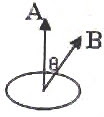## Pages

`“Life is like riding a bicycle.  To keep your balance you must keep moving.”–Albert Einstein`

## Friday, December 7, 2007

### AP Physics B&C – Equations to be Remembered in Electromagnetic Induction

Questions on electromagnetic induction are generally simple and interesting. You have to remember the following things in this section for answering the questions (especially multiple choice questions) within the stipulated time:1. Magnetic flux Ф (in weber) through a plane coil of area A (in m2) and number of turns N is given by Ф = NABcosθ where B is the magnetic flux density (in tesla or weber/m2) and θ is the angle between the vectors A and B. Note that the area vector is perpendicular to the plane of the area (fig).
If B is perpendicular to the plane of the area, the angle θ is zero and the magnetic flux is maximum, equal to NAB. If B is parallel to the plane of the area, the angle θ is 90º and the flux is zero.
2. Induced emf V(in volt) as given by Faraday’s law: V = – dФ/dt where dФ is the change in magnetic flux in a time dt.
The negative sign is because of Lenz’s law which says that the induced emf always opposes the change which produces it.
3. Induced charge (Q coulomb) flowing in a circuit: Q = Change of magnetic flux (in weber)/ Resistance (in ohm) of the circuit.
Therefore, Q = dФ/R
Note that the induced charge does not depend on the time in which the flux change occurred.
4. Motional emf (V) induced in a straight conductor of length L moving with velocity ‘v’ perpendicular to a magnetic field of flux density B is given by
5. Motional emf (voltage) induced between the ends of a straight rod of length L rotating about a perpendicular axis through one end in a magnetic field B acting perpendicular to the plane of rotation is given by
V = ½ BL2ω
where ω is the angular velocity of the rod.
If a metal disc of radius R rotates (about a central axis) with its plane perpendicular to a magnetic field B, the induced voltage between the centre and the edge of the disc is obtained by replacing L in the above expression with R so that
V =½ BR2ω
Note that the motional voltage ( 4 & 5 above) induced is numerically equal to the product of B and the area swept per second.
6. The emf induced in a plane coil of area A and number of turns N rotating with angular velocity ω in a magnetic field B acting perpendicular to the axis of rotation is given by
V = NABω sin ωt
(if the time starts when the plane of the coil is perpendicular to B).
This is an alternating emf.
If the time is reckoned from the instant the plane of the coil is parallel to the magnetic field B, the above expression gets modified as
V = NABω cos ωt
7. Ideal transformer equations:
V2/V1 = N2/N1 = I1/I2 where V1 and V2 are the primary and secondary voltages, I1 and I2 are the primary and secondary currents and N1 and N2 are the primary and secondary turns respectively.
8. Efficiency (η) of a transformer:
η = V2I2/ V1I1
Percentage efficiency is (V2I2/ V1I1) ×100.
Questions on electromagnetic induction will be discussed in the next post, following which equations as well as questions involving inductance will be discussed.